Share

# Frank solutions for Class 10 Physics chapter 3 - Sound

#### Frank Frank Class 10 Physics Part 2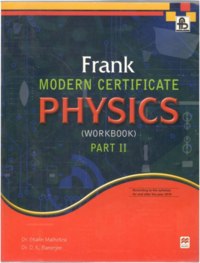## Chapter 3: Sound

Exercise 3.1Exercise 3.2Exercise

#### Chapter 3: Sound Exercise Exercise 3.1 solutions [Page 135]

Exercise 3.1 | Q 1 | Page 135
Distinguish between longitudinal and transverse waves.
Exercise 3.1 | Q 2 | Page 135
What do you understand by amplitude of an oscillation?
Exercise 3.1 | Q 3 | Page 135
Write down the relation between wavelength, frequency and wave-velocity.
Exercise 3.1 | Q 4 | Page 135
State two factors on which the velocity of a wave depends.
Exercise 3.1 | Q 5 | Page 135

How does the velocity of a wave vary with temperature?

Exercise 3.1 | Q 6 | Page 135
The speed of a wave is 350 ms-1. Find the wavelength of the wave whose frequency is 700 Hz.

#### Chapter 3: Sound Exercise Exercise 3.1 solutions [Page 136]

Exercise 3.1 | Q 1 | Page 136
Distinguish between crest and trough.
Exercise 3.1 | Q 2 | Page 136
What do you mean by wave-velocity?
Exercise 3.1 | Q 3 | Page 136
What is the nature of sound waves?
Exercise 3.1 | Q 4 | Page 136
Which has a greater speed: mechanical wave or electromagnetic wave?
Exercise 3.1 | Q 5 | Page 136
Can sound waves pass through vacuum?
Exercise 3.1 | Q 6 | Page 136
What are characteristics of a wave motion?
Exercise 3.1 | Q 7 | Page 136
Is energy transferred during wave motion?
Exercise 3.1 | Q 8 | Page 136
Distinguish between mechanical and electromagnetic waves.
Exercise 3.1 | Q 9 | Page 136
Can a transverse wave travel in air?
Exercise 3.1 | Q 10 | Page 136
Define wavelength. Give its SI units.
Exercise 3.1 | Q 11 | Page 136
What is the range of hearing for a normal person?
Exercise 3.1 | Q 12 | Page 136
Define an echo.
Exercise 3.1 | Q 13 | Page 136
What is the full form of SONAR?
Exercise 3.1 | Q 14 | Page 136
What is the full form of RADAR?
Exercise 3.1 | Q 15 | Page 136
Name the principle used to find the depth of a sea.
Exercise 3.1 | Q 16 | Page 136
What are ultrasonic?
Exercise 3.1 | Q 17 | Page 136
What are infrasonics?
Exercise 3.1 | Q 18 | Page 136
Give two applications of supersonics.
Exercise 3.1 | Q 19 | Page 136
What is the difference between echo and reverberation?
Exercise 3.1 | Q 20 | Page 136
What are the conditions necessary for the formation of echo?
Exercise 3.1 | Q 21 | Page 136
What are the lowest and highest limits of frequencies of sound which is audible to a human ear?
Exercise 3.1 | Q 22 | Page 136
Can sound waves be reflected?
Exercise 3.1 | Q 23 | Page 136
Give a simple experiment to demonstrate the reflection of sound waves.
Exercise 3.1 | Q 24 | Page 136
How will you find the velocity of sound waves?
Exercise 3.1 | Q 25.1 | Page 136

Explain how the principle of echo is used by
The bat during its flight at

Exercise 3.1 | Q 25.2 | Page 136

Explain how the principle of echo is used by

The dolphin to locate small fish as its prey

Exercise 3.1 | Q 26 | Page 136
What do you understand by sound signalling?
Exercise 3.1 | Q 27 | Page 136
What do you mean by sound ranging?
Exercise 3.1 | Q 28 | Page 136
A man fires a gun and hears its echo after 5 s. The man then moves 310 m towards the hill and fires his gun again. This time he hears the echo after 3 s. Calculate the speed of sound.
Exercise 3.1 | Q 29 | Page 136
How do bats avoid obstacles in their way, when in flight?
Exercise 3.1 | Q 30 | Page 136
State the use of echo by a bat, dolphin and fisherman.
Exercise 3.1 | Q 31 | Page 136

What is sonar? State the principle on which it is based.

Exercise 3.1 | Q 32 | Page 136
Name the waves which are used in sonar to find the depth of sea.
Exercise 3.1 | Q 33 | Page 136
A person standing between two vertical cliffs produces a sound. Two successive echoes are heard at 4 s and 6 s. Calculate the distance between the cliffs.
Exercise 3.1 | Q 34 | Page 136

Distinguish between compression and rarefaction.

#### Chapter 3: Sound Exercise Exercise 3.2 solutions [Page 145]

Exercise 3.2 | Q 1 | Page 145
What do you mean by wave motion?
Exercise 3.2 | Q 2 | Page 145
Write two differences between transverse and longitudinal waves.
Exercise 3.2 | Q 3 | Page 145
What are free vibrations?
Exercise 3.2 | Q 4 | Page 145
What is meant by the natural frequency of vibration of a body?
Exercise 3.2 | Q 5 | Page 145
What are damped vibrations?
Exercise 3.2 | Q 6 | Page 145
Explain the phenomenon of resonance giving examples.
Exercise 3.2 | Q 7 | Page 145

Fig. shows three ways in which the string of an instrument can vibrate.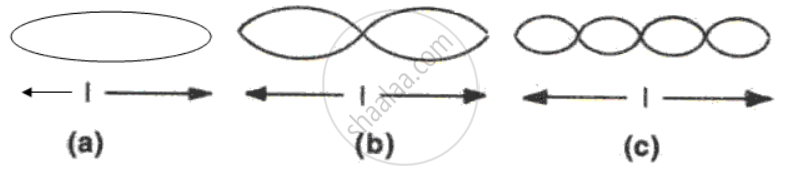(i)    Which of the diagram shows the principle note?
(ii)    Which has the frequency four times that of the first?
(iii)    What is the ratio of the frequency of the vibration in (a) and (b)?

Exercise 3.2 | Q 8 | Page 145
Distinguish between music and noise.
Exercise 3.2 | Q 9 | Page 145
Give two examples of forced vibrations.
Exercise 3.2 | Q 10 | Page 145
Differentiate between forced vibrations and resonance.
Exercise 3.2 | Q 11 | Page 145
Name three factors on which the frequency of vibration of a stretched string depends.
Exercise 3.2 | Q 12 | Page 145
State any two ways of increasing the frequency of vibration of a stretched string.
Exercise 3.2 | Q 13 | Page 145

When a troop crosses a suspension bridge the soldiers are asked to break steps. Explain the reason.

Exercise 3.2 | Q 14 | Page 145

How does the medium affect the amplitude of free vibrations of a body?

Exercise 3.2 | Q 15 | Page 145

Is pitch same as frequency?

Exercise 3.2 | Q 16 | Page 145

What adjustments would you make for tuning a stringed instrument for it to emit a note of a desired frequency?

Exercise 3.2 | Q 17 | Page 145

Explain why strings of different thicknesses are provided on a stringed instrument.
[Hint : Natural frequency of vibration of a stretched string is inversely proportional to the radius (or thickness) of string so notes of different frequencies can be produces by vibrating different strings.]

Exercise 3.2 | Q 18 | Page 145

In fig. , P, Q, R and S represent test tubes each of height 20 cm which are filled with water upto heights of 10 cm, 14 cm, 16 cm and 18 cm respectively. If a vibrating tuning fork is placed over the mouth of test tube Q, a loud sound is heard.
(i) Describe the observations with the tubes P, R and S.
(ii) Give the reason for your observation in each case.
(iii) State the principle illustrated by the above experiment.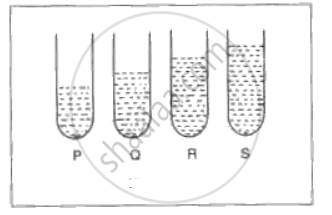#### Chapter 3: Sound Exercise Exercise 3.2 solutions [Page 146]

Exercise 3.2 | Q 1 | Page 146
With which of the following frequencies does a tuning fork of frequency 256 Hz resonate? 288 Hz, 314 Hz, 333 Hz, 512 Hz.
Exercise 3.2 | Q 2 | Page 146

In fig. shows two tuning forks P and Q of the same frequency mounted on separate sound boxes with their open ends facing each other. The fork A is set into vibration. (i) Describe your observation. (ii) State the principle illustrated by this experiment.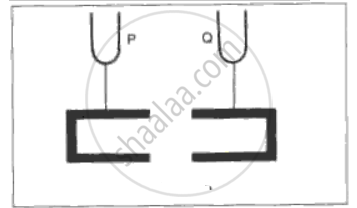Exercise 3.2 | Q 3 | Page 146

In fig. P, Q, R and S are four pendulums suspended from the same elastic string XX'. Lengths of pendulum P and S are equal, while the length of Q is smaller and R is longer. The pendulum P is set into vibration. What is your observation? Give reason for your observation.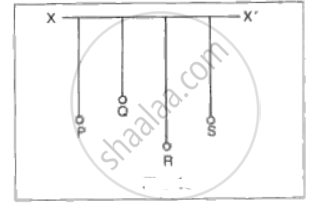#### Chapter 3: Sound Exercise Exercise solutions [Page 148]

Exercise | Q 1.1 | Page 148

Differentiate  between the following:
Light and sound waves.

Exercise | Q 1.2 | Page 148

Differentiate  between the following:

Free and forced vibrations.

Exercise | Q 1.3 | Page 148

Differentiate  between the following:

Exercise | Q 2 | Page 148
What is meant by echo? What are the conditions necessary for its formation?
Exercise | Q 3 | Page 148
Why do echoes produced in an empty auditorium usually decrease when it is full of audience?
Exercise | Q 4 | Page 148
A boy fires a gun and hears the echo 2 seconds later. If he is 480 m away from a wall, calculate the velocity of sound in air.
Exercise | Q 5 | Page 148
A girl claps and hears the echo after reflection from a cliff which is 660 m away. If the velocity of sound is 330 ms-1, calculate the time taken for hearing the echo.
Exercise | Q 6 | Page 148
A rifle shot is fired in a valley between two parallel walls. The echo from one wall is heard in 3 s and the echo from the other wall is heard 3 s later. If the velocity of sound at 00c is 330 ms-1 and the temperature in the valley is 100c, calculate the width of the valley. For every 10c rise in temperature, the velocity of sound increase by 0.61 ms-1.
Exercise | Q 7 | Page 148

Explain how is the principle of echo used by
(a) The bat during its flight at night,
(b) The dolphin to locate small fish as its prey.

Exercise | Q 8.1 | Page 148

Frequency

Exercise | Q 8.2 | Page 148

Loudness

Exercise | Q 8.3 | Page 148

Pitch

Exercise | Q 8.4 | Page 148

Quality of a musical note.

Exercise | Q 9 | Page 148
Define the terms velocity and wavelength applied to sound wave and state the relation between velocity, frequency and wavelength.
Exercise | Q 10 | Page 148
What is the wavelength of sound waves produced in air by a vibrating tuning fork whose frequency is 256 Hz when the velocity of sound in air is 330 ms-1?
Exercise | Q 11 | Page 148
State the characteristics of a musical note on which its pitch, loudness and quality depend.
Exercise | Q 12 | Page 148

In fig. shows a snap shot of a wave from of frequency 50 Hz in a string. The numbers in the diagram represents distance in centimeters. Fpr the wave motion, find
(a) Wavelength,
(b) Amplitude,
(c) Velocity.

Exercise | Q 13 | Page 148
Write down the factors on which the frequency of vibrating depends. Explain them.
Exercise | Q 14 | Page 148
What is meant by resonance? Give two examples of resonance from daily life.
Exercise | Q 15 | Page 148
Give the physical explanation for the difference between musical sounds and noises.
Exercise | Q 16 | Page 148

Name musical instrument, one in each case, which produces its notes by using the following. In each case state the method used to produce notes of different pitches and loudness.
(a) A vibrating string.
(b) A vibrating column air.
(c) Vibration of any other body.

Exercise | Q 17.1 | Page 148

Give scientific reason for the following:

Windows sometimes rattle when the low notes of a pipe organ are sounded.

Exercise | Q 17.2 | Page 148

Give scientific reason for the following:
When a nail is hammering appears to get higher and higher in pitch.

#### Chapter 3: Sound Exercise Exercise solutions [Page 149]

Exercise | Q 1 | Page 149
What is the safe limit of sound level of db for our ears?
Exercise | Q 2 | Page 149
What is the sound level for noise pollution?
Exercise | Q 3 | Page 149

What determines the pitch of a sound?

Exercise | Q 4 | Page 149
Name the subjective property of sound related to its frequency.
Exercise | Q 5 | Page 149

How is it possible to recognize a person by his voice without seeing him?

Exercise | Q 6 | Page 149

How does the wave pattern of a loud note differ from a soft note? Draw a diagram.

Exercise | Q 7 | Page 149

Why is the loudness of the sound heard by a plucked wire increased when it is mounted on a sound board?

Exercise | Q 8 | Page 149
Define the term intensity of a sound wave. State the unit in which it is measured.
Exercise | Q 9 | Page 149
How is loudness of sound related to the intensity of wave producing it?
Exercise | Q 10 | Page 149

What change do you expect in the characteristics of a musical sound if
(i) Its frequency is increased,
(ii) Its amplitude is increased?

Exercise | Q 11 | Page 149

Comment on the statement ‘loudness of sound is a subjective quantity, while intensity is an objective quantity.

Exercise | Q 12 | Page 149
State three factors on which loudness of sound heard by a listener depends.
Exercise | Q 13 | Page 149
Name the unit used to measure the sound level.
Exercise | Q 14 | Page 149
What distinguish is the sound from two musical instruments even if they are of the same pitch and same loudness?
Exercise | Q 15 | Page 149
Why do the quantities of sound of the same pitch differ when produced by different instruments?
Exercise | Q 16 | Page 149

In fig. 2 two musical notes of the same pitch and same loudness are played on a violin and on a piano. Their wave forms are as shown in the diagram below. Explain why the wave patterns are different.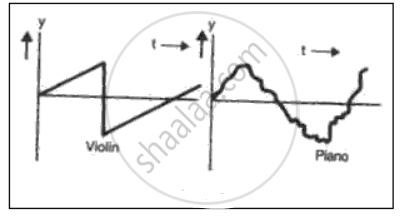## Chapter 3: Sound

Exercise 3.1Exercise 3.2Exercise

#### Frank Frank Class 10 Physics Part 2## Frank solutions for Class 10 Physics chapter 3 - Sound

Frank solutions for Class 10 Physics chapter 3 (Sound) include all questions with solution and detail explanation. This will clear students doubts about any question and improve application skills while preparing for board exams. The detailed, step-by-step solutions will help you understand the concepts better and clear your confusions, if any. Shaalaa.com has the CISCE Frank Class 10 Physics Part 2 solutions in a manner that help students grasp basic concepts better and faster.

Further, we at Shaalaa.com provide such solutions so that students can prepare for written exams. Frank textbook solutions can be a core help for self-study and acts as a perfect self-help guidance for students.

Concepts covered in Class 10 Physics chapter 3 Sound are Reflection of Sound Waves, Natural Vibrations, Damped Vibrations, Forced Vibrations and Resonance, Loudness, Pitch and Quality of Sound, Concept of Sound Numericals, Concept of Sound.

Using Frank Class 10 solutions Sound exercise by students are an easy way to prepare for the exams, as they involve solutions arranged chapter-wise also page wise. The questions involved in Frank Solutions are important questions that can be asked in the final exam. Maximum students of CISCE Class 10 prefer Frank Textbook Solutions to score more in exam.

Get the free view of chapter 3 Sound Class 10 extra questions for Physics and can use Shaalaa.com to keep it handy for your exam preparation

S